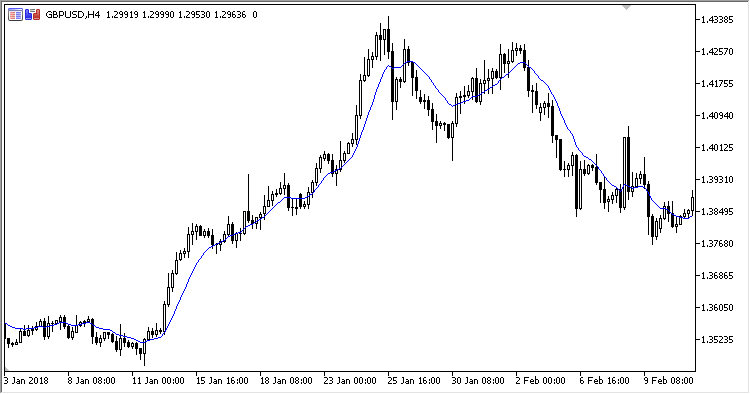Find us on Telegram!
Interesting script?
So post a link to it -
let others appraise it
You liked the script? Try it in the MetaTrader 5 terminal# GD - indicator for MetaTrader 5

|
Views:
3045
Rating:
(9)
Published:
2018.09.27 15:59

The Generalized DEMA moving average. Double exponential smoothing is applied to a price, taking into account the effect of the secondary smoothing.

The indicator has three input parameters:

• Volume factor (in percent) - secondary smoothing effect factor, in percent
• Period - calculation period
• Applied price

Calculation:

```GD = (1.0+Volume factor) * EMA1 - Volume factor * EMA2
```

where:

```EMA2 - EMA(EMA1, Period)
EMA1 - EMA(Applied price, Period)
```Translated from Russian by MetaQuotes Ltd.
Original code: https://www.mql5.com/ru/code/21832RSI_Histogram_Vol

The Relative Strength Index oscillator displayed as a color histogram, using volumes.Exp_Trading_Channel_IndexCCI_Histogram_VolInvestor_Preference_Index